# PSAT Math : How to factor a polynomial

## Example Questions

### Example Question #1 : Factoring Polynomials

What is a possible value for x in x2 – 12x + 36 = 0 ?

2

–6

There is not enough information

6

6

Explanation:

You need to factor to find the possible values for x. You need to fill in the blanks with two numbers with a sum of -12 and a product of 36. In both sets of parenthesis, you know you will be subtracting since a negative times a negative is a positive and a negative plus a negative is a negative

(x –__)(x –__).

You should realize that 6 fits into both blanks.

You must now set each set of parenthesis equal to 0.

x – 6 = 0; x – 6 = 0

Solve both equations: x = 6

### Example Question #2 : Factoring Polynomials

If r and t are constants and x2  +rx +6=(x+2)(x+t), what is the value of r?

5

7

It cannot be determined from the given information.

6

5

Explanation:

We first expand the right hand side as x2+2x+tx+2t and factor out the x terms to get x2+(2+t)x+2t. Next we set this equal to the original left hand side to get x2+rx +6=x2+(2+t)x+2t, and then we subtract x2  from each side to get rx +6=(2+t)x+2t. Since the coefficients of the x terms on each side must be equal, and the constant terms on each side must be equal, we find that r=2+t and 6=2t, so t is equal to 3 and r is equal to 5.

### Example Question #3 : Factoring Polynomials

Solve for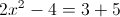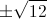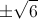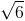Explanation:First, add 4 to both sides: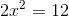Divide both sides by 2: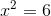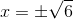### Example Question #4 : Factoring Polynomials

Letandbe integers, such that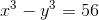. If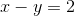and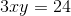, then what is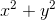?

Cannot be determined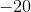Explanation:

We are told that x- y3 = 56. We can factor the left side of the equation using the formula for difference of cubes.

x- y3 = (x - y)(x2 + xy + y2) = 56

Since x - y = 2, we can substitute this value in for the factor x - y.

2(x2 + xy + y2) = 56

Divide both sides by 2.

x2 + xy + y= 28

Because we are trying to find x2 + y2, if we can get rid of xy, then we would have our answer.

We are told that 3xy = 24. If we divide both sides by 3, we see that xy = 8.

We can then substitute this value into the equation x2 + xy + y= 28.

x2 + 8 + y= 28

Subtract both sides by eight.

x2 + y= 20.

ALTERNATE SOLUTION:

We are told that x - y = 2 and 3xy = 24. This is a system of equations.

If we solve the first equation in terms of x, we can then substitute this into the second equation.

x - y = 2

x = y + 2

Now we will substitute this value for x into the second equation.

3(y+2)(y) = 24

Now we can divide both sides by three.

(y+2)(y) = 8

Then we distribute.

y2 + 2y = 8

Subtract 8 from both sides.

y2 + 2y - 8 = 0

We need to factor this by thinking of two numbers that multiply to give -8 but add to give 2. These numbers are 4 and -2.

(y + 4)(y - 2) = 0

This means either y - 4 = 0, or y + 2 = 0

y = -4, or y = 2

Because x = y + 2, if y = -4, then x must be -2. Similarly, if y = 2, then x must be 4.

Let's see which combination of x and y will satisfy the final equation that we haven't used, x- y3 = 56.

If x = -2 and y = -4, then

(-2)3 - (-4)3 = -8 - (-64) = 56. So that means that x= -2 and y = -4 is a valid solution.

If x = 4 and y = 2, then

(4)3 - 2= 64 - 8 = 56. So that means x = 4 and y = 2 is also a valid solution.

The final value we are asked to find is x2 + y2.

If x= -2 and y = -4, then x2 + y= (-2)2 + (-4)2 = 4 + 16 = 20.

If x = 4 and y = 2, then  x2 + y= (4)2 + 22 = 16 + 4 = 20.

Thus, no matter which solution we use for x and y, x2 + y= 20.

### Example Question #5 : Factoring Polynomials

How many negative solutions are there to the equation below?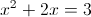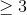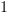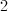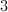Explanation:

First, subtract 3 from both sides in order to obtain an equation that equals 0: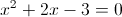The left side can be factored. We need factors of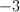that add up to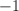andwork: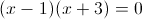Set both factors equal to 0 and solve: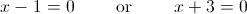To solve the left equation, add 1 to both sides. To solve the right equation, subtract 3 from both sides. This yields two solutions: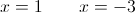Only one of these solutions is negative, so the answer is 1.

### Example Question #1 : How To Factor A Polynomial

How many of the following are prime factors of the polynomial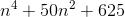?

(A)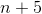(B)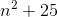(C)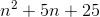(D)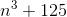None

Three

Two

One

Four

One

Explanation: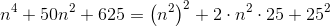can be seen to fit the pattern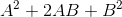:

where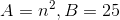can be factored as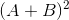, so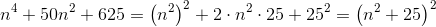, as the sum of squares, is a prime polynomial, so the complete factorization is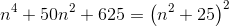,

makingthe only prime factor, and "one" the correct choice.

### Example Question #2 : How To Factor A Polynomial

Completely factor the following expression: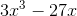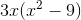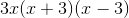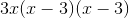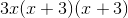Explanation:

To begin, factor out any like terms from the expression. In this case, the termcan be pulled out:Next, recall the difference of squares: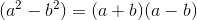Here,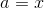and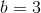..

### Example Question #6 : Factoring Polynomials

2x + 3y = 5a + 2b        (1)

3x + 2y = 4a – b           (2)

Express x– y2 in terms of a and b

–〖9a〗+ 26ab –〖3b〗2) / 5

(–9a– 27ab +3b2) / 5

–〖9a〗+ 27ab +〖3b〗2) / 5

〖–9a〗+ 26ab +〖3b〗2) / 5

(–9a– 28ab –3b2) / 5